# 宏赫化工

## 多晶和单晶氧化铝在纳米压痕过程中的变形行为和力学性能

### W. G. Mao, Y. G. Shen, C. Lu

##### The nanoscale pop-in phenomena in polycrystalline α-Al2O3 and single crystal α-Al2O3(0001) were comparatively investigated by nanoindentation with Berkovich indenters. It was found that different radii and loading rates have significant effects on the pop-ins formation, stress distributions and dislocation nucleations. Using the Hertzian contact theory and energetic model approach, the pop-in observations and resolved shear stress analysis are consistent with the explanation that the first pop-in corresponds to the nucleation of homogeneous dislocations when the theoretical shear strength is exceeded.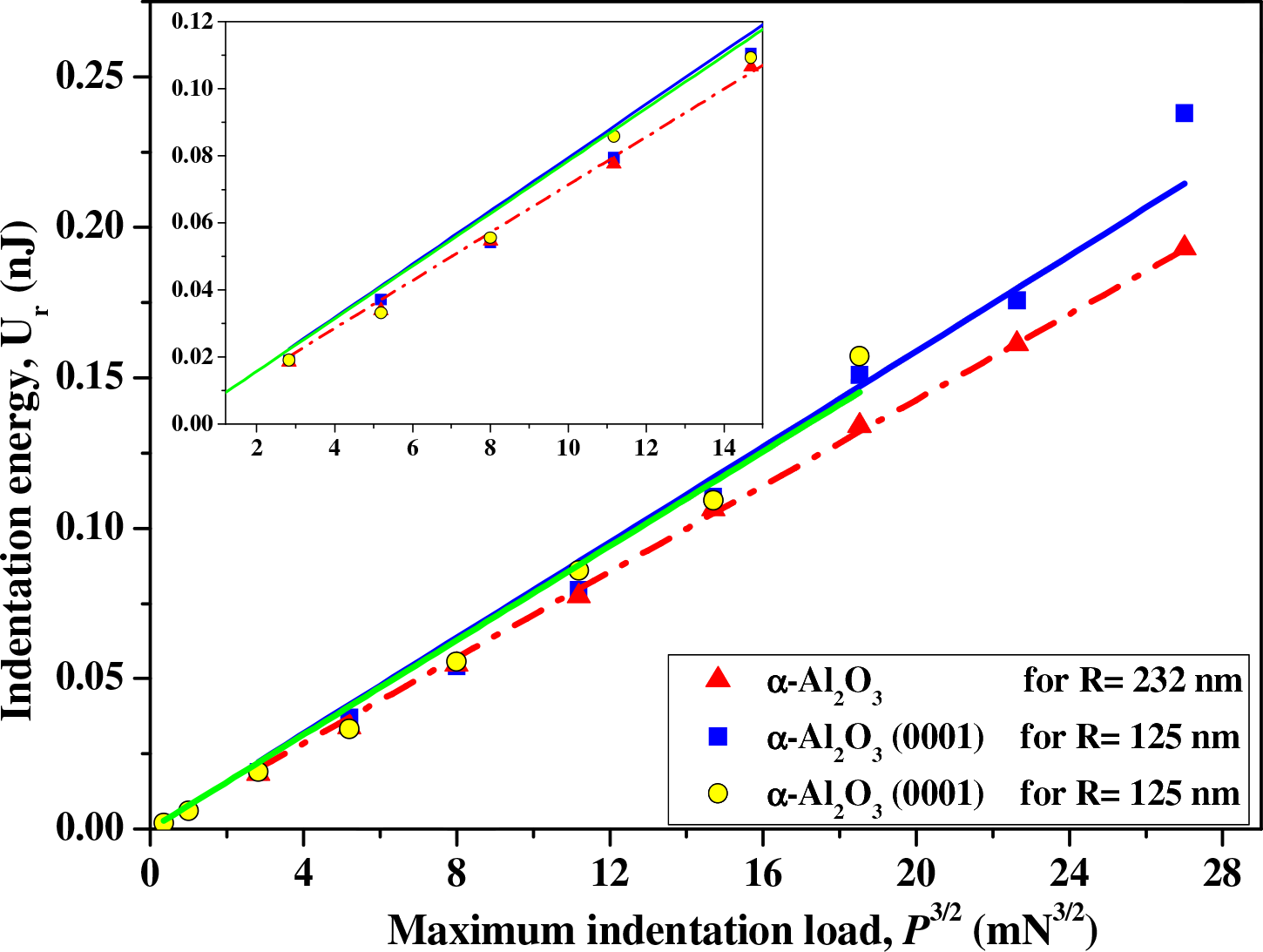Alumina (α-Al2O3) is an important oxide crystal and technologically possesses favorable physical, mechanical, and chemical properties such as high hardness and strength, high melting temperature, and excellent resistance to thermal and chemical environments. It has been extensively applied in optics, engineered components, high pressure windows, and scratch resistance devices [1, 2]. However, its technological application is somewhat limited due to poor toughness and inferior thermal shock resistance . It has been reported that Al2O3 crystals may deform plastically under very low applied loads even at room temperature [3-7]. To obtain a more detailed understanding of plastic deformation behaviors and mechanical properties, the knowledge on the elastic-plastic characteristics of Al2O3 crystals is essential . For this reason, the initial stages of elastic-plastic deformation of polycrystalline α-Al2O3 and single crystal α-Al2O3(0001) have been extensively investigated by instrumented depth-sensing nanoindentation. The techniques have been widely used as an important tool to investigate the micro-/nano-mechanical properties of small scale volumes (e.g. microelectronic devices, functional thin films, nanowires) due to the fast, precise and nondestructive merit . In previous works, single or multiple pop-in (displacement discontinuity) events were observed in α-Al2O3 and α-Al2O3(0001) crystals during nanoindentation [4-16]. However, the onset of nano-plasticity and mechanical properties are strongly influenced by factors, such as crystal orientations, applied loads, loading rates, tip radii of the indenter and temperature during tests [7, 9, 10]. The observed single or multiple pop-in events and the onset of micro-/nano-plasticity for Al2O3 crystals have been not completely understood. Thus, it is not surprising to see that there were some confusion in 3 understanding the onset of micro-/nano-plasticity and misinterpreting the plastic deformation mechanism of Al2O3 crystals.

In this paper, single or multiple pop-in behaviors and mechanical properties of the (0001) plane of single crystal α-Al2O3 by nanoindentation with a Berkovich indenter were discussed, compared with the results of polycrystalline α-Al2O3 crystal with (0001) texture . The indentation size effects (ISE) of hardness for both different crystals were found and analyzed, respectively, by the Al-Rub  and Nix-Gao  models under small size scales. The Hertzian contact theory  was used to describe the purely elastic and elastic-plastic behaviors and estimate the distributions of resolved shear stress of both crystal materials at the pop-in events. The obtained results were compared to the theoretical shear strength to establish the correlation and difference between the two crystals. According to a simple energetic approach [14-16], their mechanical properties at the first pop-in occurrence are further analyzed and compared. The new results have shed light on the understanding of the nanoscale deformation behaviors of polycrystalline and single crystal alumina.

Commercial single crystal α-Al2O3(0001) was purchased from the University Wafer Corporation. As specified by the manufacturer, these double-side polished crystals are free from defects and residual stress. Our X-ray diffraction θ−2θ measurements confirmed that α-Al2O3(0001) is the C-axis oriented high quality single crystal. The root-mean-square roughness measured by atomic force microscopy (AFM) is <0.2 nm for α-Al2O3(0001), respectively. Nanoindentation tests were performed using an instrumented nanoindenter (Triboscope, Hysitron Inc.) equipped with a three-sided pyramidal Berkovich diamond tip and an in-situ scanning probe microscopy (SPM). The force and displacement sensitivities of 4 these instruments are 100 nN and 0.2 nm, respectively. The peak loads range from 0.8 to 8 mN. For each test, the loading and unloading times were 20 s and holding time was set as 10 s at the peak load. To obtain reliable data, each cycle was repeated 8 times. Prior to nanoindentation, each indenter was carefully calibrated using standard pure fused quartz  and AFM measurements  . The detailed calibration process was given in our recent work [5, 19, 20]. The tip radius of Berkovich indenter is about 125 nm in this work.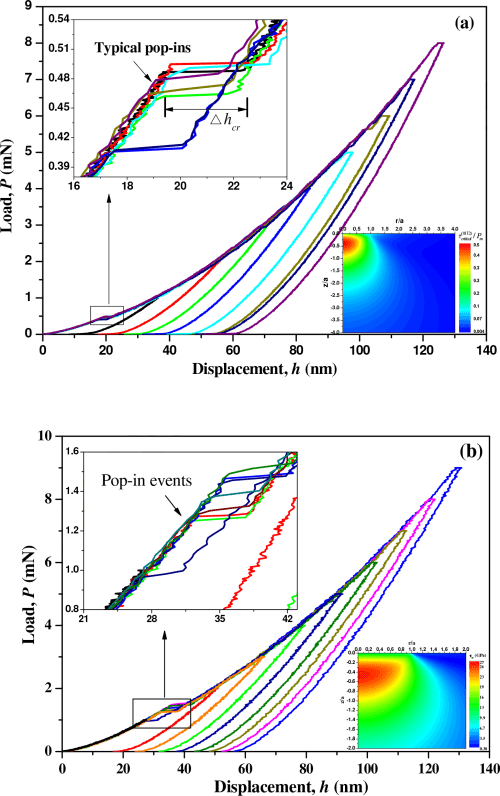Fig. 1. Typical P−h curves during nanoindentation with different size Berkovich indenters. (a) α-Al2O3(0001); and (b) α-Al2O3. The left and right insets of each figure present, respectively, the magnified pop-in phenomena and the CRSS contour with normalized coordinates at pop-in.

As shown in Fig. 1, a number of discrete bursts of pop-ins from the single crystal α-Al2O3(0001) occurred in nanoindentation [see Fig. 1(a)]. The enlargement of P−h curves in inset of Fig. 1(a) exhibits clearly a series of serrations with prominent pop-in events under low the critical pop-in indentation load Pcr values due to the initiation of dislocations [6-8]. In contrast, for the nano-grained polycrystalline α-Al2O3 crystal, the pop-in events are re-plotted [see Fig. 1(b)], where the Berkovich tip radius was estimated about 232 nm . The analysis indicate that Pcr changes from 1.2 to 1.5 mN under different loading rates. But the values of Pcr of Al2O3(0001) are much smaller in the range of 0.35−0.58 mN compared to those of polycrystalline α-Al2O3, as shown in Fig. 2(b). For single crystal α-Al2O3(0001), Pcr and the width of pop-in extension cr ∆h gradually decrease as the loading rate increase within the ~100 nm indentation depth range. However, The different loading rates seem to be a little influence on the initial plastic behavior of polycrystalline α-Al2O3 [see Fig. 2(a)].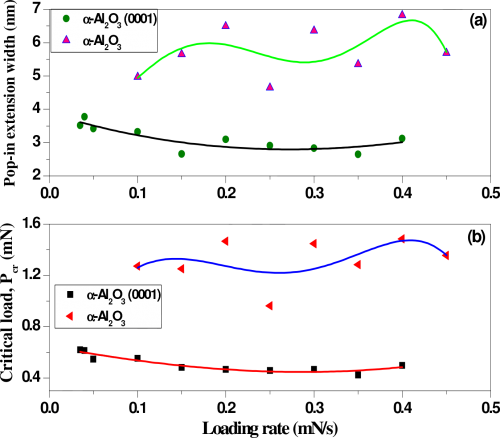Fig. 2. Effect of loading rate on the variations of Pcr and hcr during nanoindentations.

Using the method developed by Oliver and Pharr , hardness (H) and effective indentation modulus Especimen of both crystals can be estimated from the P−h curves, as shown in Fig. 3. It is interesting to observe that H displays strong indent size effect (ISE) within the range of h 100 nm, i.e. the value decreases with the increase of indentation depth. At the 5 ISE region, the average H is, respectively, 46.7 ± 15 GPa for α-Al2O3(0001) and 41.7 ± 5.0 GPa for polycrystalline α-Al2O3. At the load-independent hardness region, the range of H equals 27.5 ± 2 GPa for α-Al2O3(0001), which is in good agreement with the result of 28.9 ± 2.3 GPa . The range of H is about 30 ± 3 GPa for polycrystalline α-Al2O3. It can be concluded that H of α-Al2O3(0001) is subtle smaller than that of polycrystalline α-Al2O3 at small size scale in the present work. As we know, the ISE and large plastic strain gradient are generally based on the evolution of geometrically necessary dislocations (GNDs) and statistically stored dislocations (SSDs) beneath the indenter through the Taylor’s hardening law [11, 12]. To further discuss the ISE at small indentation depths, we attempted to modify the Al-Rub model  to analyze the hardness evolutions of α-Al2O3 crystal materials bywhere Ho is an intrinsic hardness in the limit of infinite depth and h * is a material parameter that characterizes the depth dependence of the hardness. Hy is a friction hardness.  is a material constant, whose different values implies changes in the strain history . H and h are hardness and indentation depth at a given scale. If both SSDs and GNDs are regarded as coupling in a linear form and the friction hardness is neglected during nanoindentation, i.e.  = 2 and Hy =0, the above formula changes into the Nix-Gao model . For the indentation data of polycrystalline α-Al2O3, Ho and h * are estimated as 29.3 GPa and 90.1 nm , respectively if  = 2 and Hy =0 by Eq. (1). It is interesting to find that the fitted parameters  = 2.001 and Hy = 0.01 GPa can be estimated when the two obtained Ho and h * parameters above are fixed in Eq. (1). The fitting curves by the Al-Rub model and Nix-Gao model are consistent well, as indicated in Fig. 3(a). However, for α-Al2O3(0001) crystal, Ho is assumed to equal the 6 average hardness (27.5 GPa) at the non-ISE region. It is found that the fitted curve by the Al-Rub model is far better than that by the Nix-Gao model because of the too small indentation depth of h < 100 nm. Moreover, the fitted parameter h * by the Al-Rub model is equal to about 55 nm, which agree well with the experimental results [see Fig. 3(a)].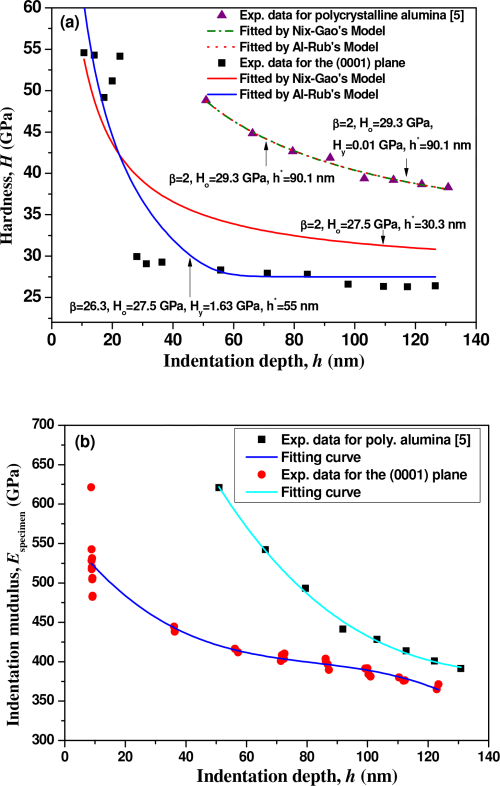Fig. 3. Comparison of H and Especimen of α-Al2O3 and α-Al2O3(0001). (a) hardness; (b) indentation modulus.

The values of effective indentation modulus Especimen for polycrystalline α-Al2O3 and α-Al2O3(0001) can be estimated from the reduced indentation modulus Er data measured by the nanoindentation instrument. Here, 1/Er = (1− 2 specimen v )/Especimen + (1−vi 2 )/Ei , where Ei = 1141 GPa and νi = 0.07 are Young’s modulus and Poisson’s ratio of a diamond indenter , and νspecimen = 0.28 is Poisson’s ratio of polycrystalline alumina . Especimen for polycrystalline α-Al2O3 is greater than that for α-Al2O3(0001), especially at h < 100 nm. All values gradually decrease with the increase of h and eventually go to a saturation state [see Fig. 3(b)]. The mean Especimen values of 466 ± 20 GPa obtained from α-Al2O3 at the steady state is comparable to 392 ± 44 GPa obtained by Ruppi et al.  and ~458 GPa by Twigg et al. . The average Especimen of 420.6 ± 20 GPa from α-Al2O3(0001) is consistent well with 431 GPa reported by Swadener , 441 ± 4.7 GPa measured by Oliver  and 444 ± 20.7 GPa studied by Ruppi .

To further study the mechanical properties of nano-grained α-Al2O3 and single crystal α-Al2O3(0001), another essential ductility parameter, the so-called true hardness Htrue, was calculated on the basis of the energy principle of indentation contacts proposed by Sakai . The relationship between the hysteresis loop energy Ur and the maximum indentation load 3/2 Pmax is used to calculate Htrue,where α0 = 3 3 and ψ = 67.3° are a geometrical constant and the semi apex angle of a Berkovich indenter , respectively. In the case of elastic/plastic surface deformation, the loop energy Ur can be calculated by the area difference between loading and unloading paths. Ur and its corresponding Pmax present reasonable fits to straight lines, as shown in Fig. 4. Their values of true hardness can be determined by the slope of the linear relationship. The Htrue of α-Al2O3 is 73.4 GPa under 232 nm indenter tip, and the Htrue of α-Al2O3(0001) is 59.2 and 60.7 GPa by 125 nm tip. These values are in excellent agreement with the result of Htrue= ~ 61 GPa for α-Al2O3(0001) during nanoindentation reported by Nowak et al. .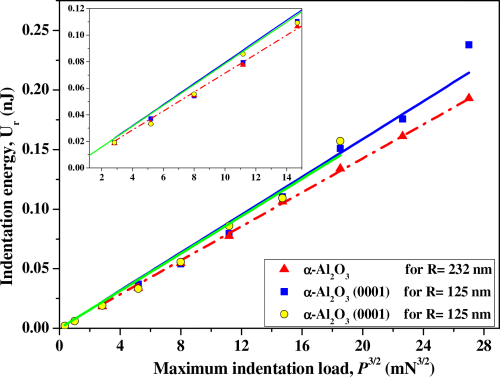Fig. 4. The relationship between Ur and 3/2 Pmax for α-Al2O3 and α-Al2O3(0001) under different indenter tip radii. The inset is magnified from the small indentation load regime.

The most significant feature of the first pop-in represents the transition from purely elastic to elastic/plastic regimes. To further clarify and compare the elastic characteristics between α-Al2O3 and Al2O3(0001), additional nanoindentation tests with the maximum loads of 1.0 and 0.3 mN were performed below Pcr (i.e., 1.3 N and 0.35 mN, respectively). The inset in Fig. 5(a) indicates that there is no hysteresis in the P–h cycles, revealing a perfectly elastic behavior. In the tests, the maximum indentation depth is only 28 nm, so the Berkovich indenter tip can be approximately considered as spherical . Based on the elastic Hertzian theory, the P−h relationship is generally described as 3/2 1/2 4 / 3 P r = h R E . The elasticity of the two crystals can be predicted by fitting the P−h curves with the P ~ h 1.5 function form, that is, P = 0.00665h 1.5 and P = 0.005767h 1.5 [see inset in Fig. 5(a)], where the units of load P and displacement h are mN and nm, respectively.Fig. 5. Comparison of typical nanoscale elastic and elastic/plastic features for α-Al2O3 and α-Al2O3(0001) under nanoindentation. (a) The inset displays the purely elastic P-h curves of the two crystals, which fits well with the predictions of the Hertzian contact theory. (b) The linearly relationship of Pcr and cr ∆h of the two crystals at pop-ins.

To perform better visibility and clear analysis of the nano-plasticity onsets for α-Al2O3 and α-Al2O3(0001), extra nanoindentation tests were carried out by increasing the maximum loads up to 2.0 and 0.8 mN, respectively. It is seen from Fig. 5(a) that the first irreversible 8 pop-ins are observed during loading, which can be regarded as the threshold for dislocation nucleation in the almost defect-free region. A significant difference is found between the two crystals. The α-Al2O3 shows a larger pop-in under a 232 nm indenter tip. However, for the first pop-in events of α-Al2O3(0001) under 125 nm indenter tip, Pcr, the critical indentation depth hcr and ∆hcr are smaller than those corresponding values of α-Al2O3. To visually compare the pop-in features, the relationships of Pcr and ∆hcr are plotted in Fig. 5(b). In the case of polycrystalline α-Al2O3, all data points fall on a straight line. The indent data obtained from α-Al2O3(0001) also represent the similar line form.

The critical resolved shear stress (CRSS) at which pop-in occurs usually approaches to the theoretical shear strength of the indented material . Here, the Hertzian contact theory was used to evaluate the CRSS of the two crystals and then to compare with their theoretical shear strengths. Contour plots of the CRSS at the experimentally observed pop-in loads for α-Al2O3 and α-Al2O3(0001) are shown in inset of Fig. 1, respectively. According to the Hertzian analysis, for isotropic polycrystalline α-Al2O3 in Fig. 1(b), the maximum shear stress τmax at pop-in is τmax = 0.31P0, where 2 3 2 1/3 0 (6 / ) r p = PE π R . τmax locates at the point with z/a = 0.5 and r/a = 0 and its value is 27.8 ± 2.0 GPa. However, for anisotropic α-Al2O3(0001), the primary slip system was estimated as the pyramidal slip {01 12}< 2021 > when indenting on the (0001) plane. For the slip plane (0112) , the maximum shear stress of 28.8 GPa locates underneath the indenter of coordinates (r / a = 0, z / a = −0.405) [see inset in Fig. 1(a)]. The detailed analysis are given in other paper . The shear moduli G values of α-Al2O3 and α-Al2O3(0001) are 170 and 150 GPa [27-29], respectively. It is interesting to note that both τmax values are in the reasonable range of their theoretical shear strengths (~G/30 to 9 G/5) . On a month to month basis, it was found that the first pop-in events of these two crystals were readily observed and very reproducible in such a way that Pcr is approximately proportional to the ∆hcr , indicating that the stored elastic energy before the first pop-in event may be partially dissipated through the collective dislocation nucleation and motion. Thus, the experimentally observed pop-in data and CRSS analysis are consistent, revealing that the first pop-ins correspond to the nucleation of homogeneous dislocations when the theoretical shear strength is exceeded.

The onset of elastic-plastic transition of alumina crystals are assumend to be attributed to slip and the nucleation of homogeneous dislocations under such a small indentation load and depth. To evaluate the prismatic dislocation loop radii for α-Al2O3 and α-Al2O3(0001) at pop-ins, the procedure used by Gouldstone et al.  was employed. According to a simple energetic model for dislocation emission during nanoindentation, we have the energy relationship below by considering an overall energy balance between the elastic energy, the dislocations interaction energy and the dislocations self-energy,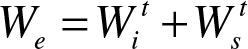where We is the total elastic energy under the loading curve up to the pop-in,Using several simplifying assumptions, Gouldstone et al. expressed the interaction energy t Wi of the dislocation loops created by the indentation as ,where b is the burger vector, Rd is the dislocation loop radius at pop-in, and ρ is the dislocation loop spacing that approximately equals burger vector b . The total self-energy 10 term t Ws is given as,where N is the number of dislocation loops punched out beneath the indenter and N ≈ ∆hcr/b. In equations (3) to (6), G and v are elastic constants of α-Al2O3 and α-Al2O3(0001), b, N and ρ can be determined from the slip direction, where b = 0.476 nm [28, 29, 31]. Pcr , hcr , and ∆hcr can be directly obtained from nanoindentation data. Since the only unknown quantity in equations (5) and (6) is the radii Rd of the dislocation loops, which can be obtained by solving equation (3). From Fig. 1(a), Pcr ≈ 1.25−1.48 mN, hcr ≈ 32.0−35.8 nm, and ∆hcr ≈ 5.9−6.7 nm, the corresponding dislocation loop radius of α-Al2O3 is Rd = 3.2 ± 0.2 nm. Similarly, from Fig. 1(b), Pcr ≈ 0.36−0.58 mN, hcr ≈ 16.7−21.0 nm, and ∆hcr ≈ 3.3−3.8 nm, the corresponding dislocation loop radius of α-Al2O3(0001) is Rd = 1.1 ± 0.2 nm. It is noted that Rd of α-Al2O3 at pop-ins is 65.5 % larger than that of α-Al2O3(0001).

In summary, we present the new results of nanoscale pop-in phenomena and mechanical properties of single crystal α-Al2O3(0001) and nano-grained polycrystalline α-Al2O3 by nanoindentation. Hardness for both crystals exhibit strong ISE at small indentation depth range of ~ 100 nm. Using the Hertzian contact theory, contour plots of the CRSS at the experimentally observed pop-ins for the two crystals show that the maximum resolved shear stress occurs at a different position beneath the contact surface for each crystal [CRSS = 27.8 GPa for α-Al2O3 and CRSS = 28.8 GPa α-Al2O3(0001), respectively]. The linearly relationship between Pcr and ∆hcr is contributed to the collective dislocation nucleation during nanoindentation. By energetic considerations, the dislocation loop radii are determined to be ~3.2 and ~1.1 nm for α-Al2O3 and α-Al2O3(0001), respectively. The magnitude of the indenter tip radius affects the critical indentation loads of the first pop-in, CRSS distribution and dislocation nucleation.

Highlights

The new results of nanoscale pop-in phenomena and mechanical properties of single crystal α-Al2O3(0001) and nano-grained polycrystalline α-Al2O3 by nanoindentation were discussed, considering loading rates and indenter tip radii. Hardness for both single crystal α-Al2O3(0001) and nano-grained polycrystalline α-Al2O3 exhibit strong ISE at small indentation depth range of ~ 100 nm. The contour plots of the CRSS at the experimentally observed pop-ins for the two crystals show that the maximum resolved shear stress occurs at a different position beneath the contact surface for each crystal [CRSS = 27.8 GPa for α-Al2O3 and CRSS = 28.8 GPa α-Al2O3(0001), respectively]. By energetic considerations, the dislocation loop radii are determined to be ~3.2 and ~1.1 nm for α-Al2O3 and α-Al2O3(0001), respectively. The magnitude of the indenter tip radius affects the critical indentation loads of the first pop-in, CRSS distribution and dislocation nucleation.